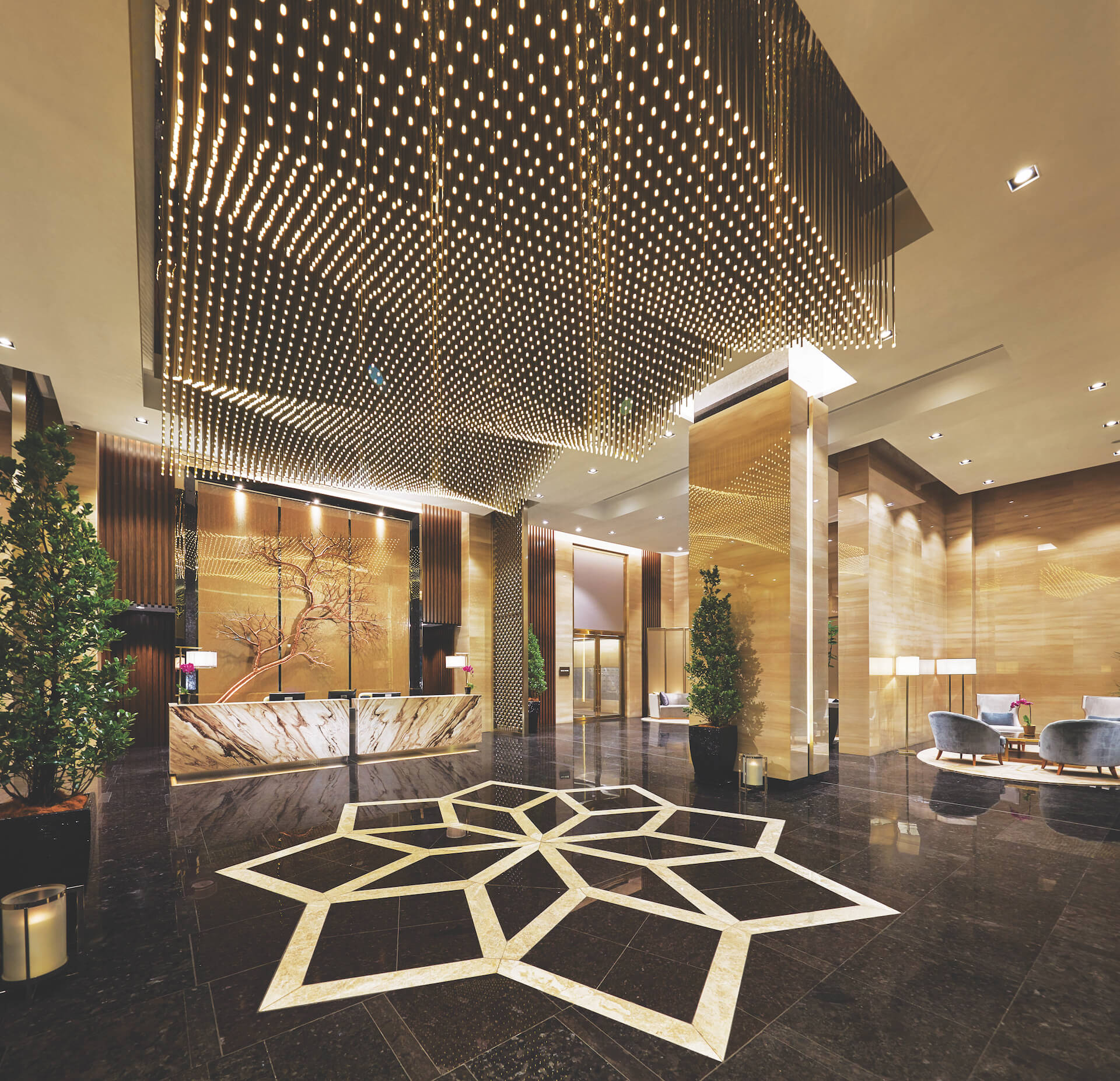the

# privilege of ownership

when you step in
at the sky lobby (level 9)when you step in

• v

• v

at the sky lobby
(level 9)

• v

• v

• v

• v

• v

• v

• v

• v

• v

• v

• v

• v

• v

• v

• v

• v

• v

• v

• v

• v

• v

• v

• v

• v

• v